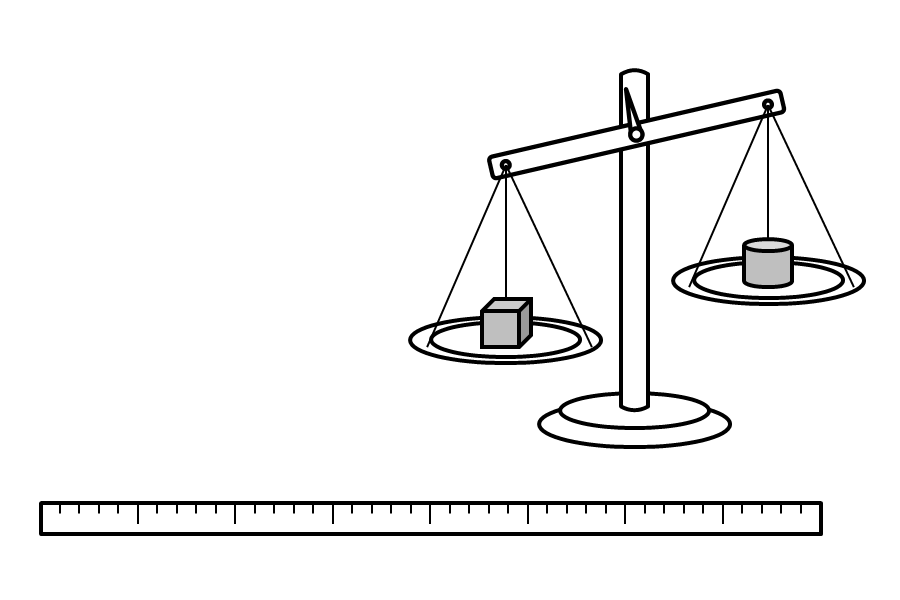## Learn These Words First (6H)

6-28. compare, compares, to compare, comparing, compared, compare one with another.

[You compare J and K.] = You think about J and K, and you try to know more about them. You think about the things you know about J and K that are the same or different. You think about what you can say about J more than or less than you can say about K.

[I compared one machine with the other machine.]

[After I compared the machines, I chose the one that was less heavy.]

6-29. weight, weights, weight of, weights of.

[The weight of J is the same as the weight of K.] = You know this about J and you know the same thing about K: You know J is not more heavy than K, and K is not more heavy than J.

[The weight of this big animal is more than the weight of this small animal.]

6-30. measure, measures, to measure, measuring, measured.

[You measure X.] = You do something to know the length of X, or the weight of X, or something else about X. Then you can compare this with what you know about other things. You can compare numbers if you can count what you know about X and other things, like this: If you want to know the weight of X, you can use small things that all have the same weight, and count the number you need to make a group that has the same weight as X. Then you can count the number of these small things that you need to make a group that has the same weight as the other thing you want to compare. And then you can compare the numbers.

[You can measure these two things to know if one is long or big or heavy more than the other.]These two things can be used to measure other things.

The long thing can be used to measure the length of something.

The machine can be used __________.